Home > CC2MN > Chapter cc22 > Lesson cc22.2.1 > Problem2-39

2-39.

Copy these incomplete number lines. Label the missing numbers on each of them. Homework Help ✎

1.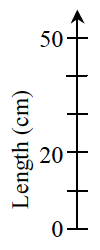Use the information on the number line to determine the value of each segment.

There are $5$ segments that make $50$ cm. How much is each segment?

Each segment is $10$ cm.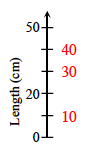1.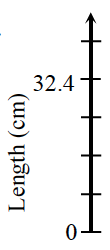See part (a).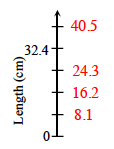1.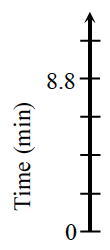Four segments represent $8.8$ minutes. How much is each segment?

1.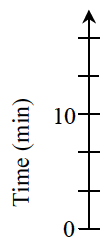See part (a).